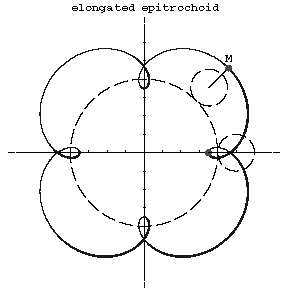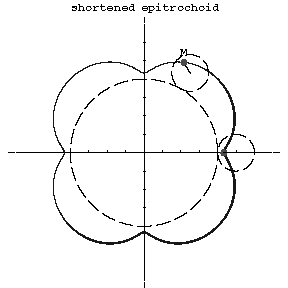# Trochoid

A plane curve that is the trajectory of a point $M$ inside or outside a circle that rolls upon another circle. A trochoid is called an epitrochoid (Fig.1a, Fig.1b) or a hypotrochoid (Fig.2a, Fig.2b), depending on whether the rolling circle has external or internal contact with the fixed circle.Figure: t094330aFigure: t094330bFigure: t094330cFigure: t094330d

The parametric equations of the epitrochoid are:

$$x=(R+mR)\cos mt-h\cos(t+mt),$$

$$y=(R+mR)\sin mt-h\sin(t+mt);$$

and of the hypotrochoid:

$$x=(R-mR)\cos mt+h\cos(t-mt),$$

$$y=(R-mR)\sin mt-h\sin(t-mt),$$

where $r$ is the radius of the rolling circle, $R$ is the radius of the fixed circle, $m=R/r$ is the modulus of the trochoid, and $h$ is the distance from the tracing point to the centre of the rolling circle. If $h>r$, then the trochoid is called elongated (Fig.1a, Fig.2a), when $h>r$ shortened (Fig.1b, Fig.2b) and when $h=r$, an epicycloid or hypocycloid.

If $h=R=r$, then the trochoid is called a trochoidal rosette; its equation in polar coordinates is

$$\rho=a\sin\mu\phi.$$

For rational values of $\mu$ the trochoidal rosette is an algebraic curve. If $R=r$, then the trochoid is called the Pascal limaçon; if $R=2r$, an ellipse.

Trochoids are related to the so-called cycloidal curves (cf. Cycloidal curve). Sometimes the trochoid is called a shortened or elongated cycloid.

How to Cite This Entry:
Trochoid. Encyclopedia of Mathematics. URL: http://encyclopediaofmath.org/index.php?title=Trochoid&oldid=42492
This article was adapted from an original article by D.D. Sokolov (originator), which appeared in Encyclopedia of Mathematics - ISBN 1402006098. See original article